Скачать презентацию PHY 2049 Physics II n Tutoring Center is

• Количество слайдов: 11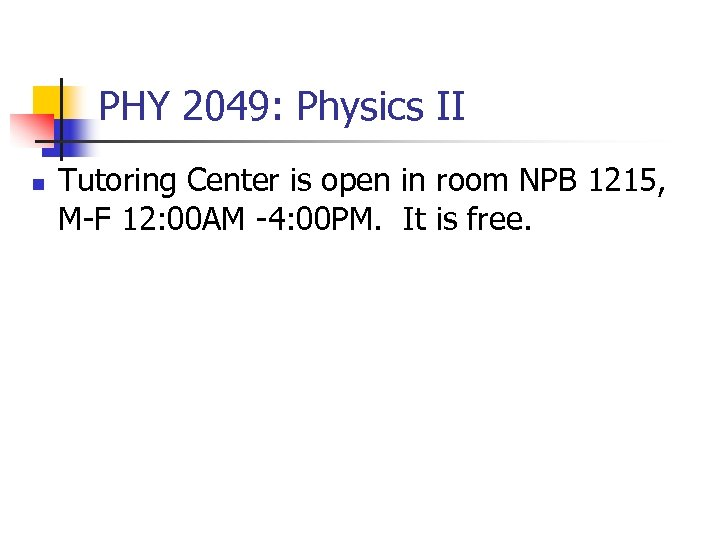PHY 2049: Physics II n Tutoring Center is open in room NPB 1215, M-F 12: 00 AM -4: 00 PM. It is free.HITT 1 You buy a “ 75 W” light bulb. The label means that: A. no matter how you use the bulb, the power will be 75 W B. the bulb was filled with 75 W at the factory C. the actual power dissipated will be much higher than 75 W since most of the power appears as heat D. the bulb is expected to burn out after you use up its 75 W E. none of the above n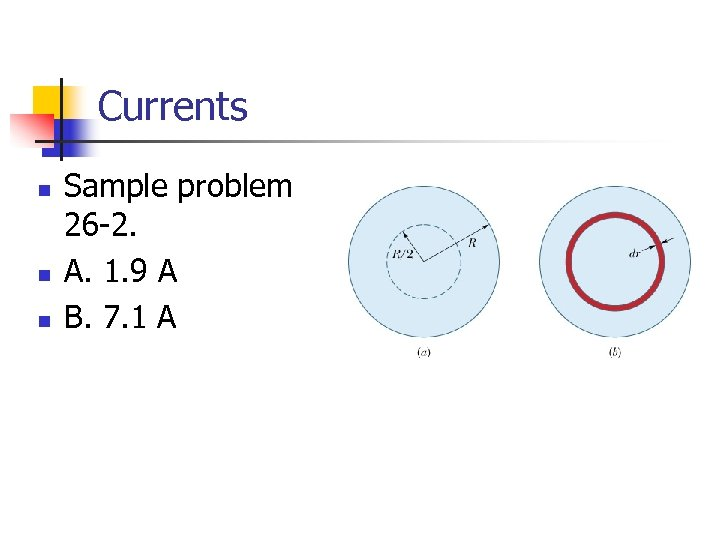Currents n n n Sample problem 26 -2. A. 1. 9 A B. 7. 1 A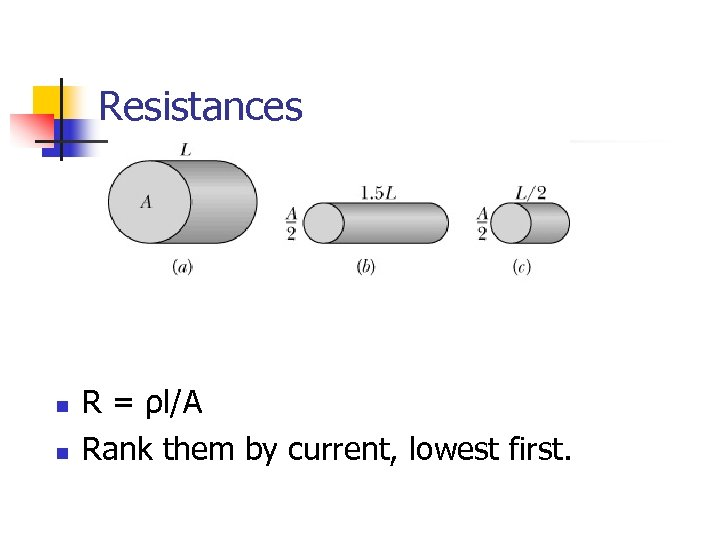Resistances n n R = ρl/A Rank them by current, lowest first.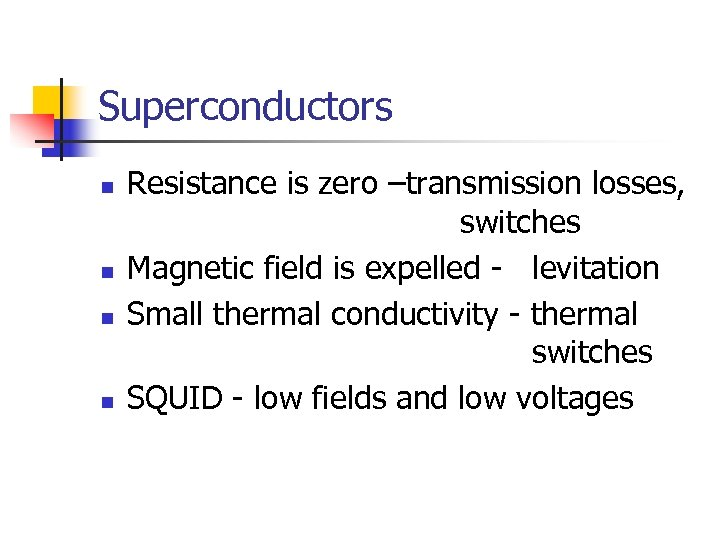Superconductors n n Resistance is zero –transmission losses, switches Magnetic field is expelled - levitation Small thermal conductivity - thermal switches SQUID - low fields and low voltages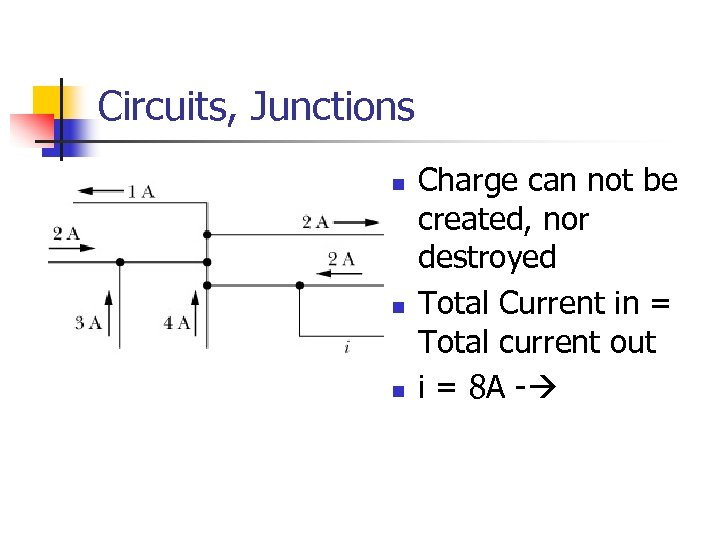Circuits, Junctions n n n Charge can not be created, nor destroyed Total Current in = Total current out i = 8 A -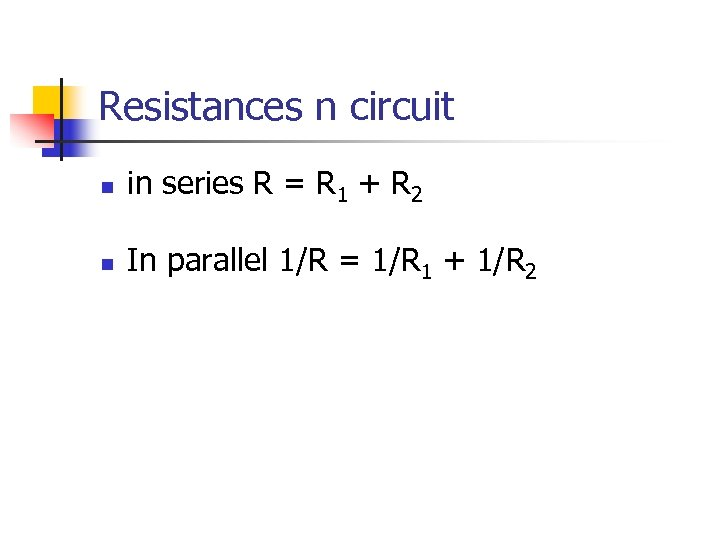Resistances n circuit n in series R = R 1 + R 2 n In parallel 1/R = 1/R 1 + 1/R 2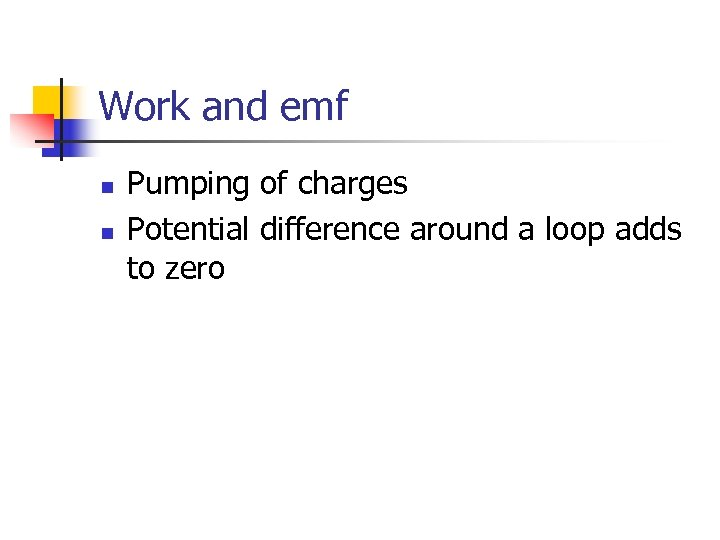Work and emf n n Pumping of charges Potential difference around a loop adds to zero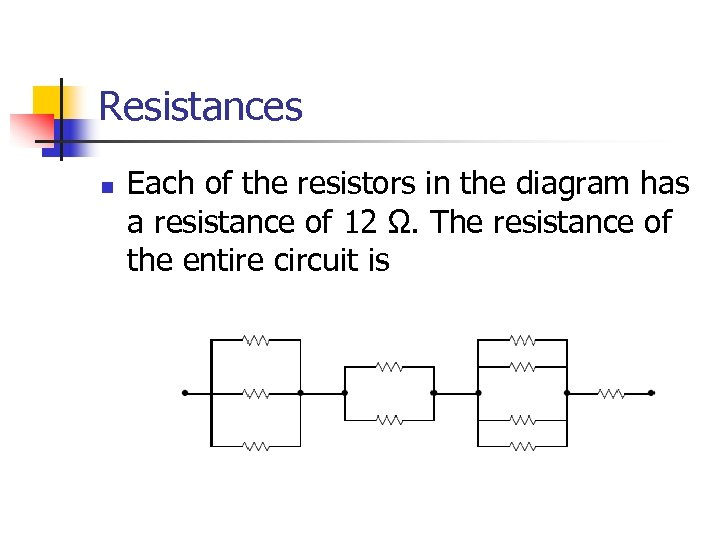Resistances n Each of the resistors in the diagram has a resistance of 12 Ω. The resistance of the entire circuit is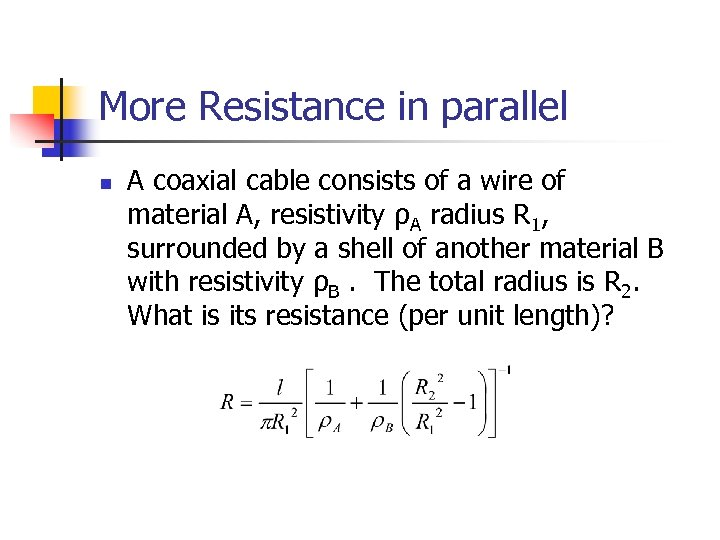More Resistance in parallel n A coaxial cable consists of a wire of material A, resistivity ρA radius R 1, surrounded by a shell of another material B with resistivity ρB. The total radius is R 2. What is its resistance (per unit length)?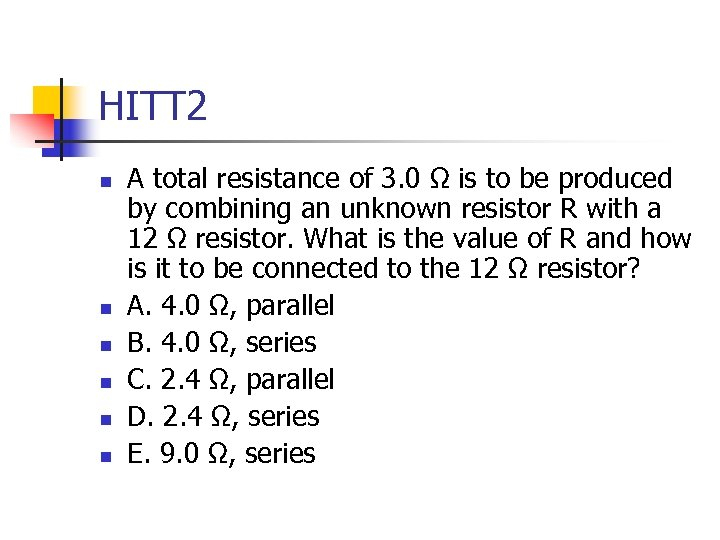HITT 2 n n n A total resistance of 3. 0 Ω is to be produced by combining an unknown resistor R with a 12 Ω resistor. What is the value of R and how is it to be connected to the 12 Ω resistor? A. 4. 0 Ω, parallel B. 4. 0 Ω, series C. 2. 4 Ω, parallel D. 2. 4 Ω, series E. 9. 0 Ω, series